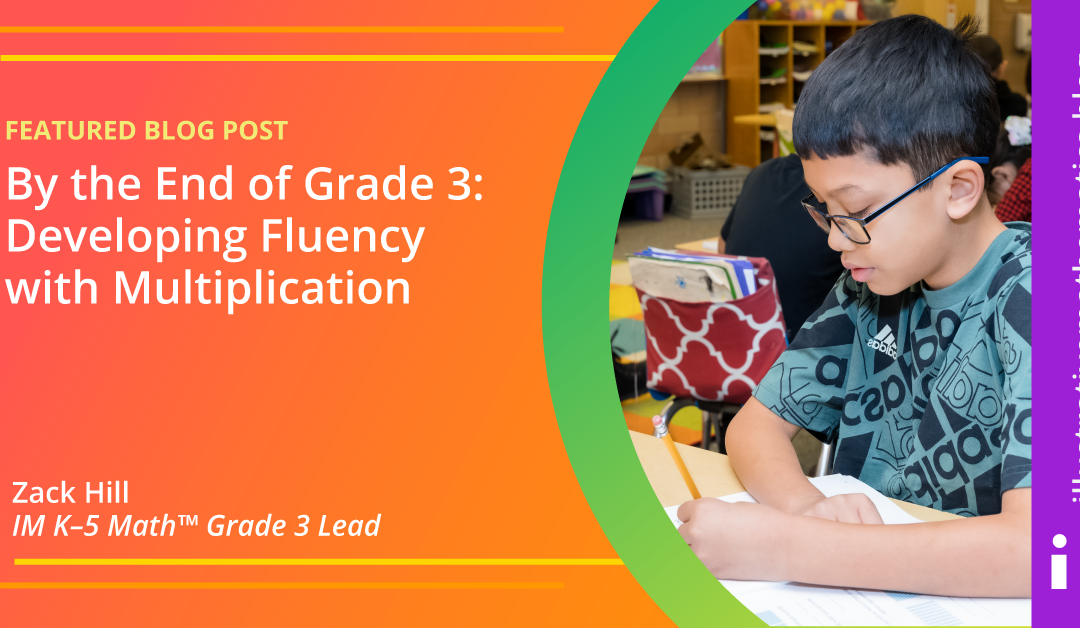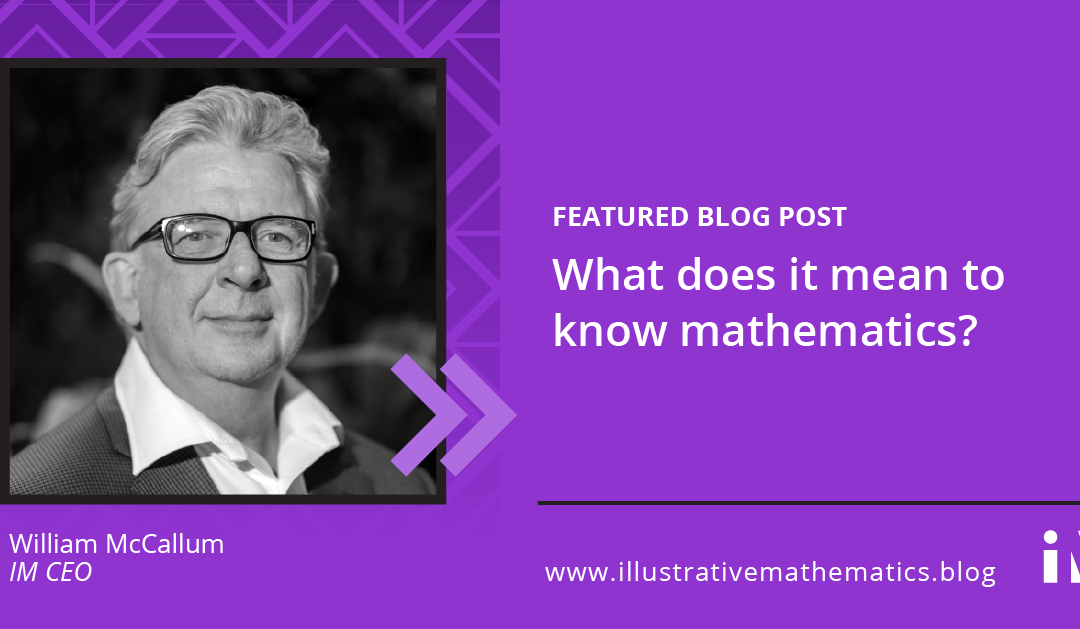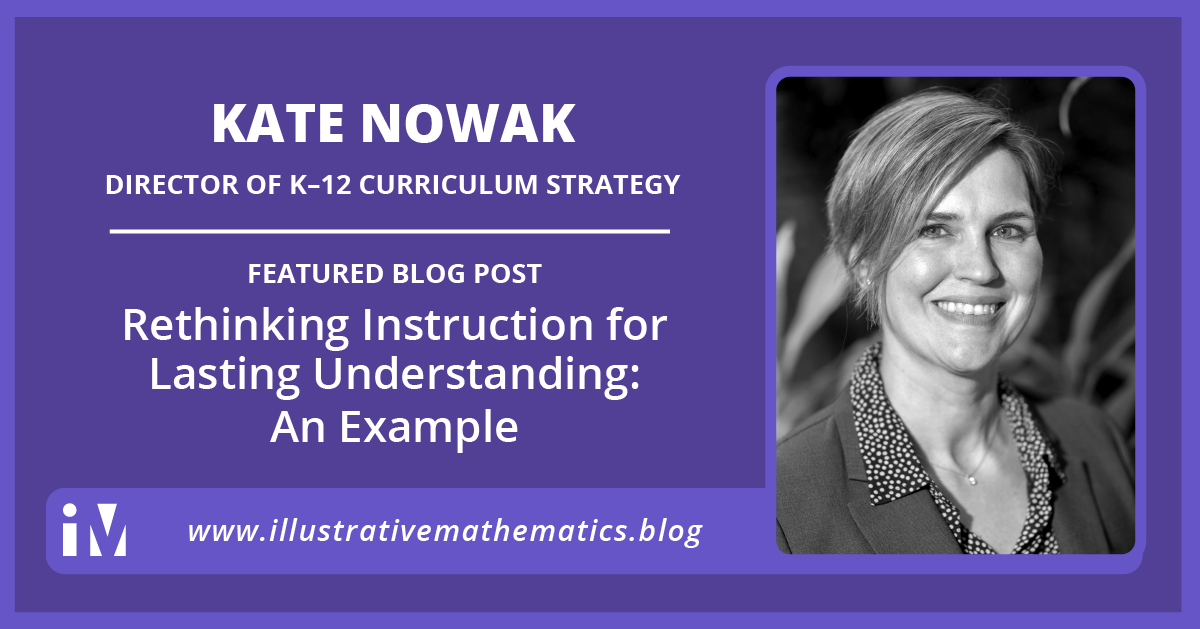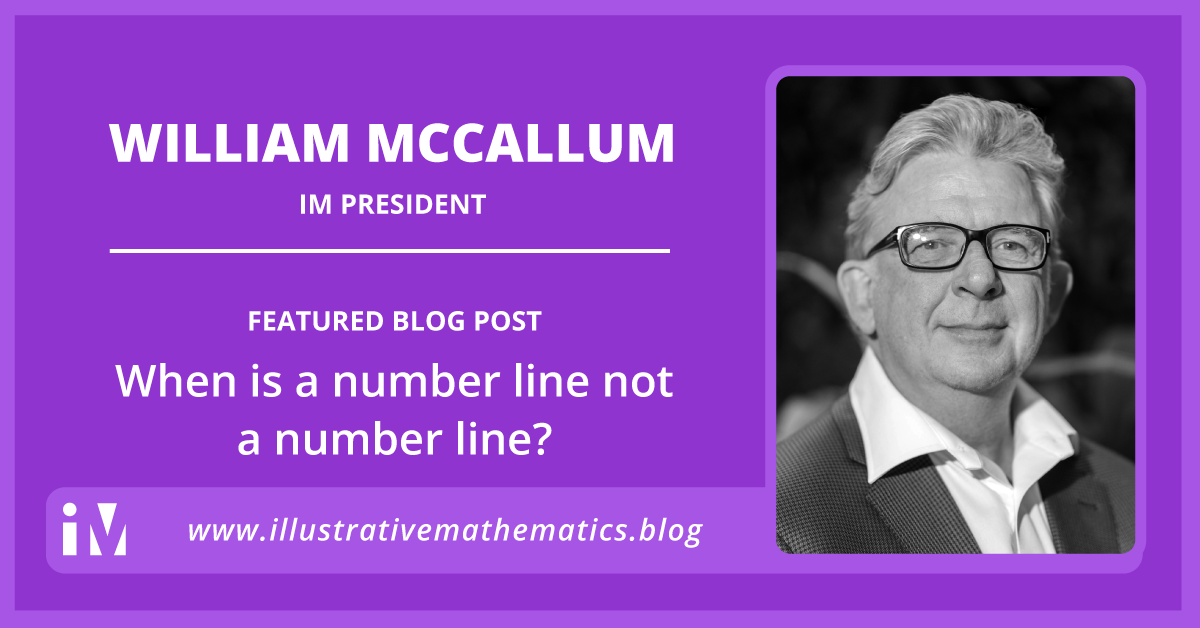## By the End of Grade 3: Developing Fluency with Multiplication

By Zack Hill The major work of grade 3 includes representing and solving problems that involve multiplication and division. Then, by the end of grade 3, students are expected to know from memory all products of two one-digit numbers. What does this look like and how...## What does it mean to know mathematics?

By William McCallum A world where all learners know, use, and enjoy mathematics. Perhaps the most mysterious verb in the IM vision—a world where all learners know, use, and enjoy mathematics—is the first one: know.  Knowing mathematics means being able to “do...## Rethinking Instruction for Lasting Understanding: An Example

By Kate Nowak How do we help our students build mathematical understandings that endure past the unit test? If we want students to construct strong, reliable bases of mathematical knowledge, our instruction needs to do more than present explicit procedures—even when...## When is a number line not a number line?

By William McCallum The number line is a seemingly simple object: a straight line with two points marked 0 and 1. Those two points are the seeds of great complexity, however. Whole numbers are located at positions marked off by iterating the interval. Fractions are...## Ratio Tables are not Elementary

By William McCallum In grade 3, as students start to learn about multiplication, they think about products like 6 x 7 in terms of equal groups. 6 x 7 is the number of things when you have 6 groups with 7 things in each group. They might start out calculating that...